Next: About this document ... Up: lab_template Previous: Area Approximations

# Exercises

1. Consider the area under the curve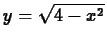on the interval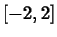.
1. Use the formula for the area of a circle to compute the exact value of this area.
2. Using the left endpoint rule, find the minimum number of subintervals required to approximate this area to within a tolerance of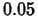. That is find the value of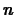such that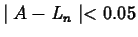, but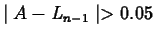.

2. Consider the function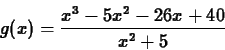on the interval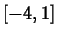.
1. Use the error bound formula to find the smallest value ofthat guarantees that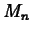approximates the area to within. That is, find the smallest value ofthat guarantees that.

2. The value ofgiven by the error bound is usually conservative. That is, in practice the desired accuracy can be achieved with a smaller value of. Given that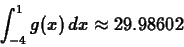find the smallest value ofsuch thatand compare it to the value you obtained in the previous exercise.

3. Repeat the previous exercise, but use the left endpoint rule. That is, find the smallest value ofsuch that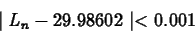Your value ofshould be much larger than the one in the previous exercise.

4. The midpoint rule is usually a much better approximation to the area than either the left endpoint or right endpoint rules. However, suppose you approximate the area by taking the average of the values given by the left endpoint or right endpoint rules. That is, we define a new approximationby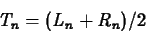Using the same function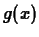and the same interval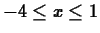, find the smallest value ofsuch that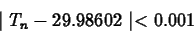Is this value ofcloser to the one you found for the midpoint rule or the one you found for the left endpoint rule? Does this make sense to you?Next: About this document ... Up: lab_template Previous: Area Approximations
Dina Solitro
2001-01-12